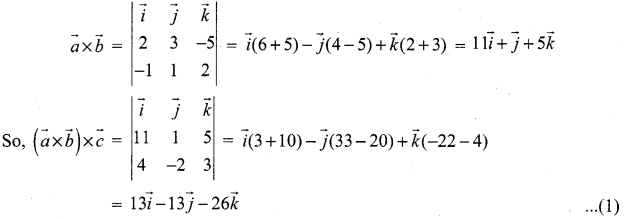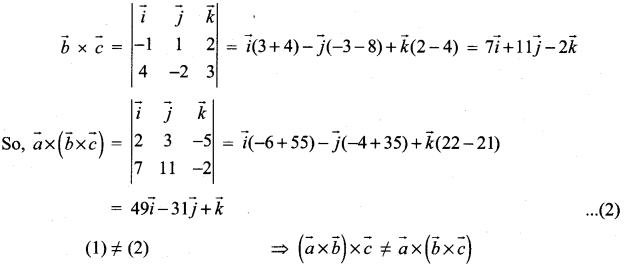# Samacheer Kalvi 12th Maths Solutions Chapter 6 Applications of Vector Algebra Ex 6.2

You can Download Samacheer Kalvi 12th Maths Book Solutions Guide Pdf, Tamilnadu State Board help you to revise the complete Syllabus and score more marks in your examinations.

## Tamilnadu Samacheer Kalvi 12th Maths Solutions Chapter 6 Applications of Vector Algebra Ex 6.2

Question 1.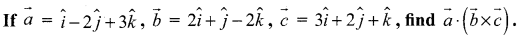Solution: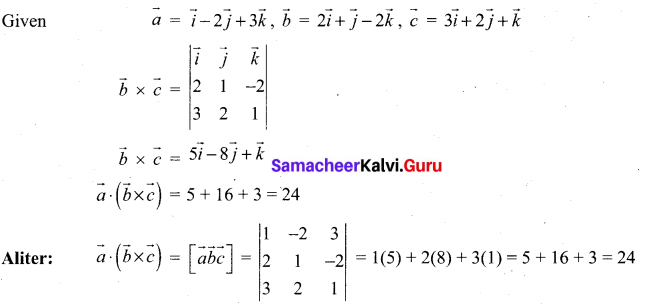Question 2.
Find the volume of the parallelepiped whose coterminous edges are represented by the vectors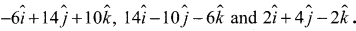.
Solution:
Volume of the parallelepiped = $$\| \vec{a}, \vec{b}, \vec{c}]$$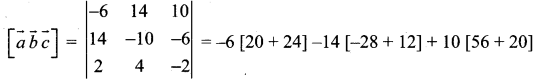= -264 + 224 + 760 = 720 cubic unitsQuestion 3.
The volume of the parallelepiped whose coterminus edges are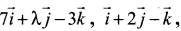, $$-3 \vec{i}+7 \vec{j}+5 \vec{k}$$ is 90 cubic units. Find the value of λ
Solution:
Given, Volume of the parallelepiped = 90 cubic units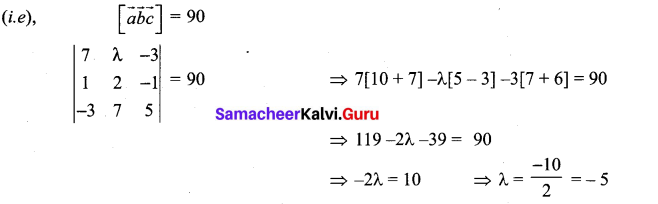Question 4.
If $$\vec{a}, \vec{b}, \vec{c}$$ are three non-coplanar vectors represented by concurrent edges of a parallelepiped of volume 4 cubic units, find the value of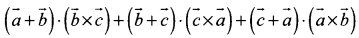Solution:
Let $$\vec{a}, \vec{b}, \vec{c}$$ be the concurrent edges of parallelepiped
Given volume of parallelepiped = 4 cubic units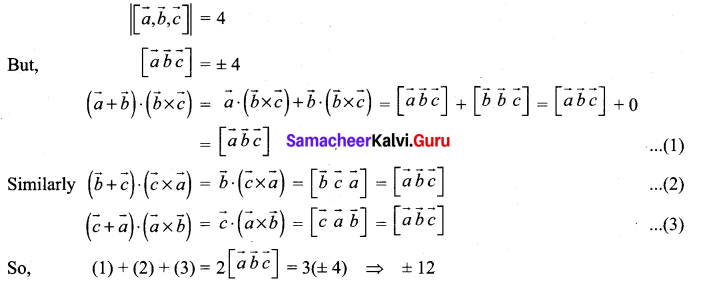Question 5.
Find the altitude of a parallelepiped determined by the vectors $$\vec{a}=-2 \hat{i}+5 \hat{j}+3 \hat{k}$$, $$\hat{b}=\hat{i}+3 \hat{j}-2 \hat{k}$$ and $$\vec{c}=-3 \vec{i}+\vec{j}+4 \vec{k}$$ if the base is taken as the parallelogram determined by b and c.
Solution:
Volume = Base Area × Height
$$|[\vec{a}, \vec{b}, \vec{c}]|=|\vec{b} \times \vec{c}|$$ × Height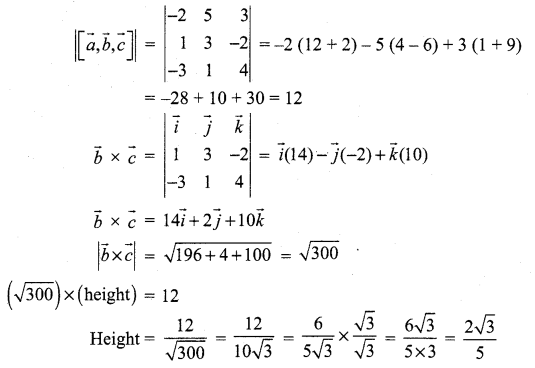Question 6.
Determine whether the three vectors $$2 \hat{i}+3 \hat{j}+\hat{k}, \hat{i}-2 \hat{j}+2 \hat{k}$$ and $$3 \hat{i}+\hat{j}+3 \hat{k}$$ are coplanar.
Solution: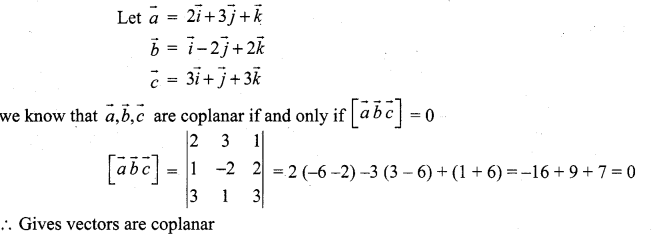Question 7.
Let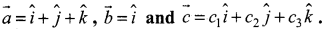If c1 = 1 and c2 = 2, find c3 such that $$\vec{a}, \vec{b}$$ and $$\vec{c}$$ and c are coplanar.
Solution: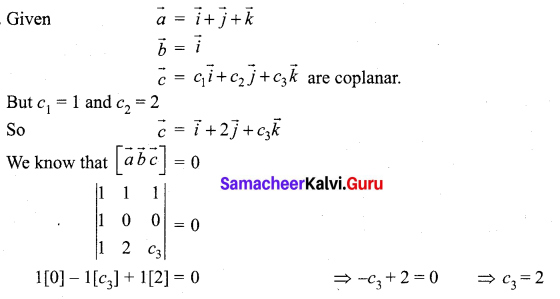Question 8.
If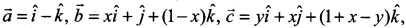, show that $$[\vec{a} \vec{b} \vec{c}]$$ depends neither x nor y.
Solution: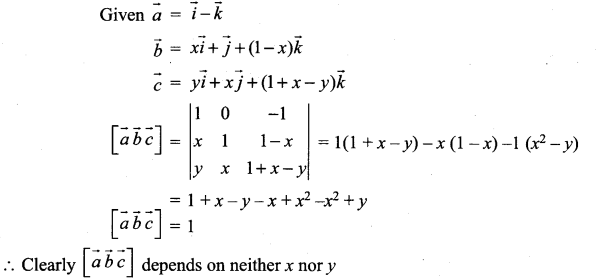Question 9.
If the vectors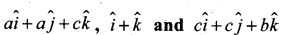are coplanar, prove that c is the geometric mean of a and b.
Solution: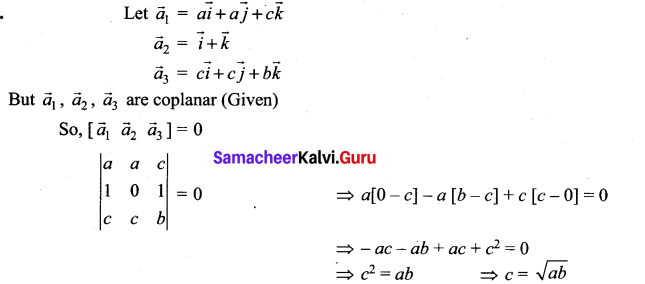∴ c is the geometric means of ‘a’ and ‘b’.

Question 10.
Let $$\vec{a}, \vec{b}, \vec{c}$$ be three non-zero vectors such that $$\vec{c}$$ is a unit vector perpendicular to both $$\vec{a}$$ and $$\vec{b}$$. If the angle between $$\vec{a}$$ and $$\vec{b}$$ is $$\frac{\pi}{6}$$ show that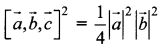.
Solution: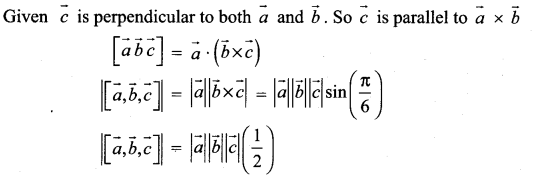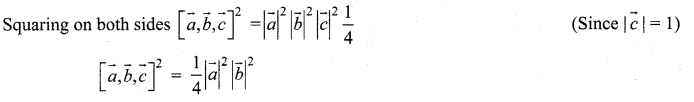### Samacheer Kalvi 12th Maths Solutions Chapter 6 Applications of Vector Algebra Ex 6.2 Additional Problems

Question 1.
If the edges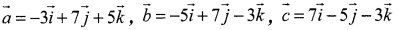meet a vertex, find the volume of the parallelepiped.
Solution:
Volume of the parallelepiped =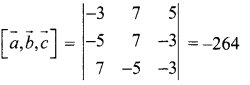The volume cannot be negative
∴ Volume of parallelepiped = 264 cu. units

Question 2.
If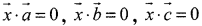and $$\vec{x} \neq \overrightarrow{0}$$ then show that $$\vec{a}, \vec{b}, \vec{c}$$ are coplanar.
Solution: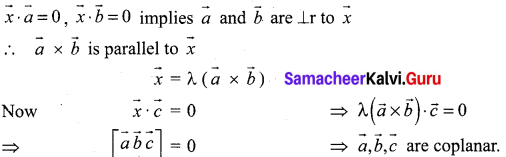Question 3.
The volume of a parallelepiped whose edges are represented by $$-12 \vec{i}+\lambda k$$, $$3 \vec{j}-\vec{k}, 2 \vec{i}+\vec{j}-15 \vec{k}$$ is 546. Find the value of λ.
Solution:
Volume of the parallelepiped =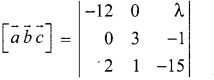= -12 [-45 + 1] – 0 () + λ [0 – 6] = -12 (-44) -6 λ
= 528 – 6λ = 546 (given)
⇒ -6λ = 546 – 528 = 18
∴ λ = $$\frac{18}{-6}$$ = -3

Question 4.
Prove that $$|\vec{a} \vec{b} \vec{c}|$$ = abc if and only if $$\vec{a}, \vec{b}, \vec{c}$$ are mutually perpendicular.
Solution: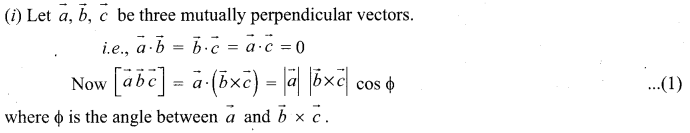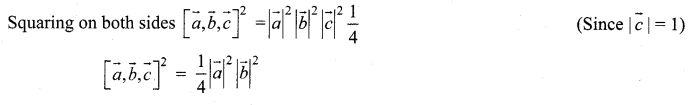Question 5.
Show that the points (1, 3, 1), (1, 1, -1), (-1, 1, 1), (2, 2, -1) are lying on the same plane.
Solution: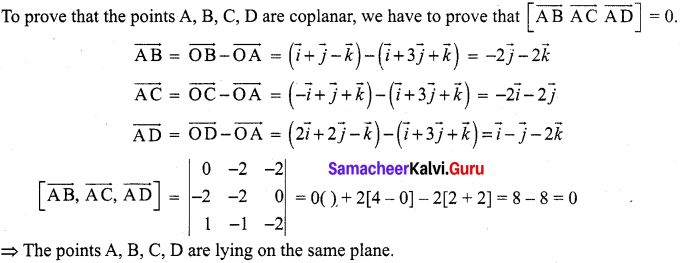Question 6.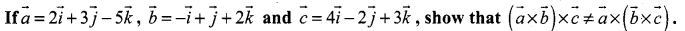Solution: